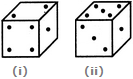# Non Verbal Reasoning - Cubes and Dice - Discussion

### Discussion :: Cubes and Dice - Section 2 (Q.No.19)

19.

Two positions of a cube are shown below. When the number 4 will be at the bottom, then which number will be at the top?[A]. 3 [B]. 5 [C]. 6 [D]. Cannot be determined

Explanation:

Number 1 is common to both the positions of the dice. We assume the dice in fig. (ii) to be rotated so that 1 remains on the RHS face (i.e. face II as per activity 1) and the numbers 5 and 3 move to the faces hidden behind 2 and 4 respectively (in fig. (i). Then, clearly 3 lies opposite 4. Hence, when 4 is at the bottom then 3 mist lie on the top.